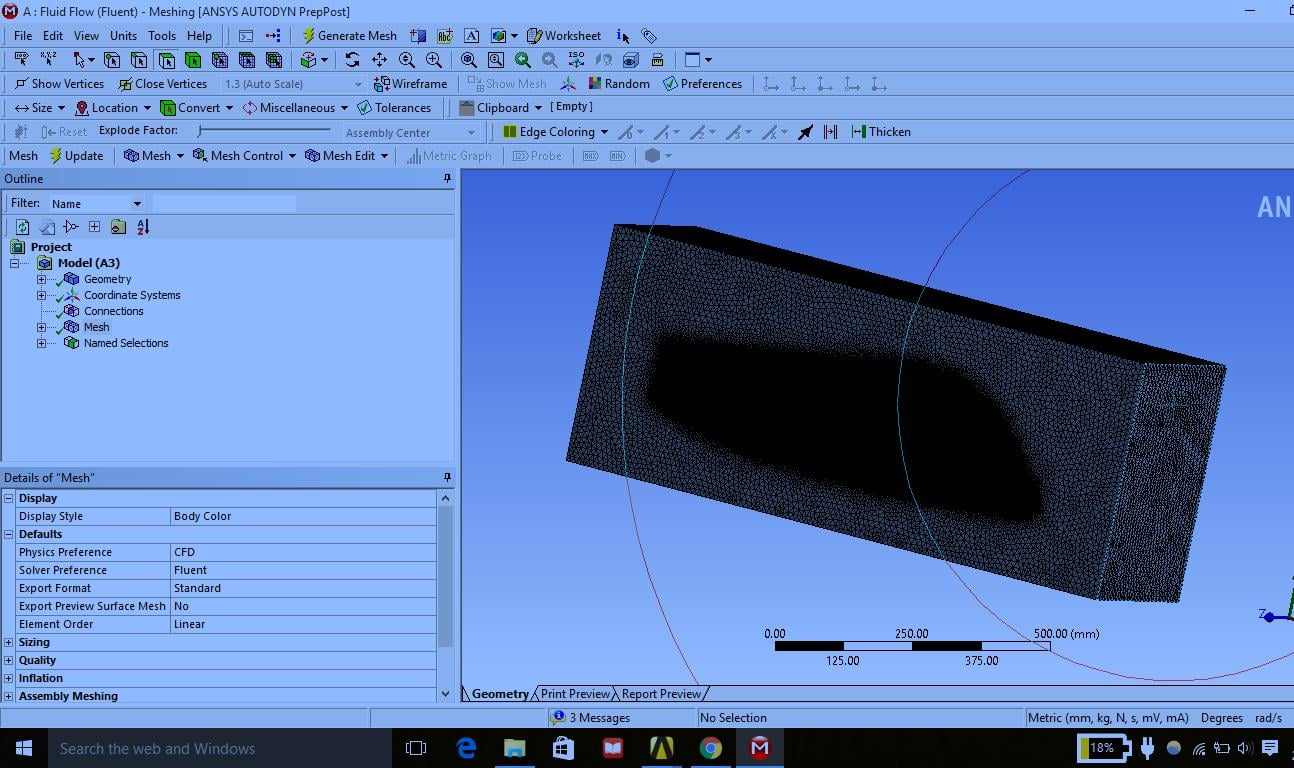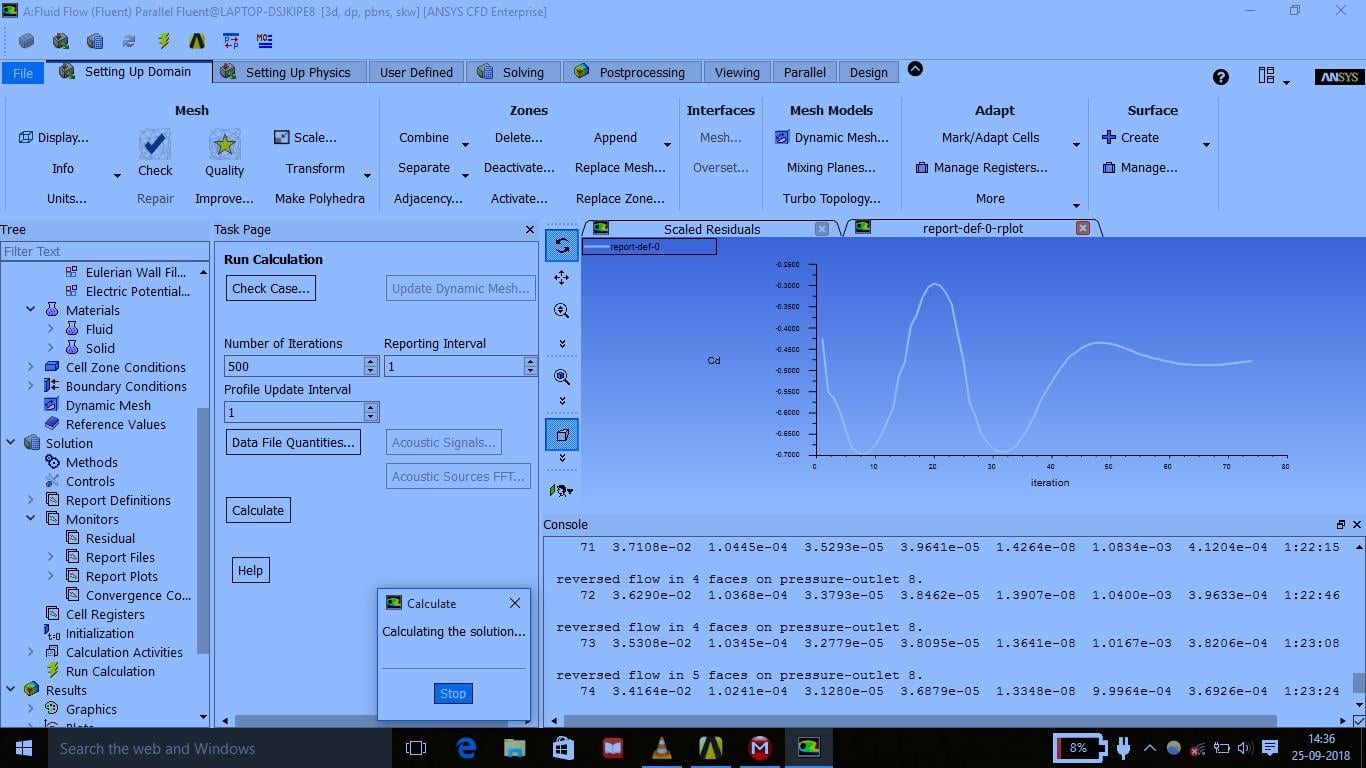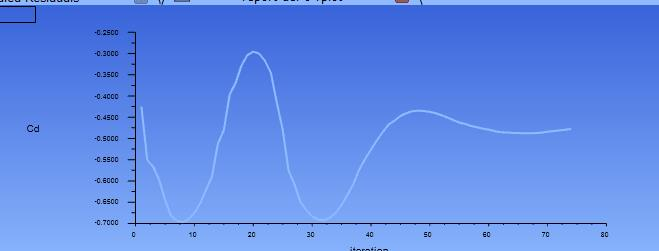## Fluids

•mstricks
Subscriber

####This is a fuselage and i want calculate drag coefficient of this body I have done all the procedures but my drag coefficient is coming negative.

I request to please guide me what parameters i need to set

to calculate drag coefficient.

Thanks

•paguado
Ansys Employee

Have you checked the drag force? Is it negative or positive? The drag coefficient is obtained from this force and may take the sign. You can also use 1/2*rho*S*U_inf^2 to non-dimensionalize the drag Force and take the net value to calculate the Cd. It would be good to know if this absolute value is the same as the one you observe when you plot the Cd

•DrAmine
Ansys Employee

Check the direction vector along which drag force and the coefficients are calculated. The vector for drag will be the direction of the flow (for lift will be perpendicular for that direction). paguado wrote what you can else try!

•mstricks
Subscriber

Have you checked the drag force? Is it negative or positive? The drag coefficient is obtained from this force and may take the sign. You can also use 1/2*rho*S*U_inf^2 to non-dimensionalize the drag Force and take the net value to calculate the Cd. It would be good to know if this absolute value is the same as the one you observe when you plot the Cd

thanks sir I understand what you are saying